# 【C++】类和对象（中）(万字详解)1.🚀构造函数

1.😯定义

2.😯特征

2.🚀默认构造函数

3.🚀析构函数

1.😯概念

2.😯特性

4.🚀拷贝构造函数

1.😯概念

2.😯特性

5.🚀运算符重载

1.😯为什么要重载运算符

2.😯赋值运算符重载格式

3.😯加法的实现

6.🚀赋值运算符重载

1.😯赋值运算符重载格式

2.😯拷贝构造和赋值重载

7.🚀<<和>>运算符重载

8.🚀const修饰的成员函数

1.😯const修饰的权限问题

2.😯取地址重载

🏠总结

# 前言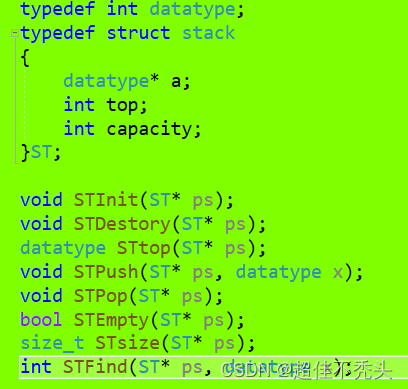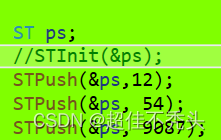# 一、🏠类的六个默认成员函数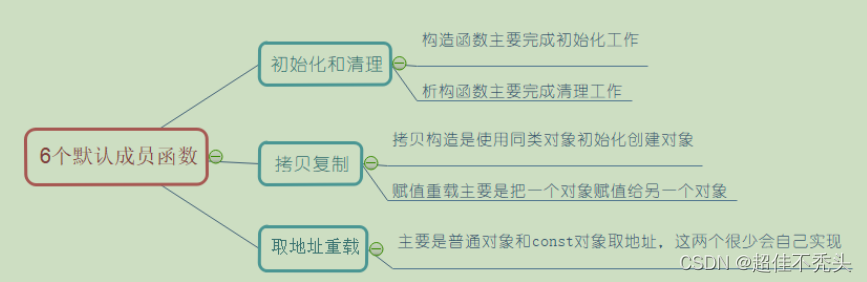## 1.🚀构造函数

### 1.😯定义

构造函数，他不是真正去构造一个函数，只是向我们上面所说的，去初始化一个对象。

``````class Date
{
public:
void Init(int year, int month, int day)
{
_year = year;
_month = month;
_day = day;
}

void Print()
{
cout << _year << "-" << _month << "-" << _day << endl;
}

private:
int _year;
int _month;
int _day;
};
int main()
{
Date d1;
d1.Init(2022, 7, 5);
d1.Print();
Date d2;
d2.Init(2022, 7, 6);
d2.Print();

return 0;
}``````

对于Date类，可以通过 Init 公有方法给对象设置日期，但如果每次创建对象时都调用该方法设置 信息，未免有点麻烦，那能否在对象创建时，就将信息设置进去呢？

``````

class Date
{
public:
//构造函数
Data(int year, int month, int day)
{
_year = year;
_month = month;
_day = day;
}
......
};

int main()
{
Date d1(2022, 7, 5);
d1.Print();
Date d2(2022, 7, 6);
d2.Print();

return 0;
}``````

### 2.😯特征

构造函数可以重载（重载（点击链接有详细解释），函数名相同，参数不同就构成重载）

``````  Date(int year, int month, int day)
{
_year = year;
_month = month;
_day = day;
}

Date()
{
_year = 0;
_month = 0;
_day = 0;
}

Date(int year=1, int month=1, int day=1)
{
_year = year;
_month = month;
_day = day;
}``````

``````int main()
{
Date d1(2022, 7, 5);
Date d2(2022, 7, 6);
// 无参数时，要这样调用，不可以Data d3();，会与函数声明发生冲突
//，就相当于 Data func();，是错误的
Date d3;

return 0;
}``````

``````class Date
{
public:
void Print()
{
cout << _year << "-" << _month << "-" << _day << endl;
}

private:
int _year;
int _month;
int _day;
};
int main()
{
Date d1;
d1.Print();
}``````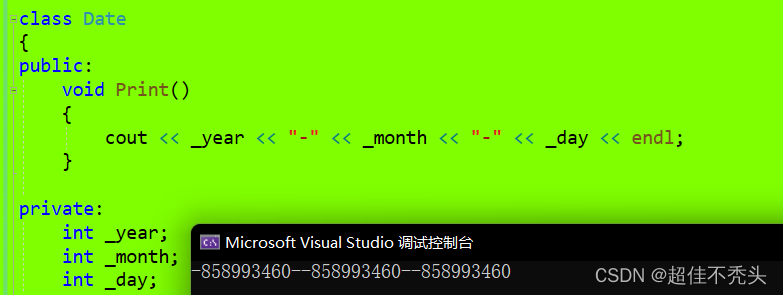``````class Stack
{
public:
Stack(int capacity = 4)
{
cout << "Stack(int capacity = 4)" << endl;

_a = (int*)malloc(sizeof(int)*capacity);
if (_a == nullptr)
{
perror("malloc fail");
exit(-1);
}

_top = 0;
_capacity = capacity;
}

void Push(int x)
{
// ....
// 扩容
_a[_top++] = x;
}

private:
int* _a;
int _top;
int _capacity;
};

class MyQueue {
public:
void push(int x)
{
_pushST.Push(x);
}

Stack _pushST;
Stack _popST;

//size_t _size;
};
``````

``````class MyQueue {
public:
void push(int x)
{
_pushST.Push(x);
}

Stack _pushST;
Stack _popST;

size_t _size=0;
};
``````

## 2.🚀默认构造函数

``````class Stack
{
public:
Stack(int capacity = 4)
{
cout << "Stack(int capacity = 4)" << endl;

_a = (int*)malloc(sizeof(int)*capacity);
if (_a == nullptr)
{
perror("malloc fail");
exit(-1);
}

_top = 0;
_capacity = capacity;
}

void Push(int x)
{
// ....
// 扩容
_a[_top++] = x;
}

private:
int* _a;
int _top;
int _capacity;
};

class MyQueue {
public:
void push(int x)
{
_pushST.Push(x);
}

Stack _pushST;
Stack _popST;

size_t _size=0; //这里不是初始化，是缺省值
};

int main()
{
MyQueue q;
return 0;
}``````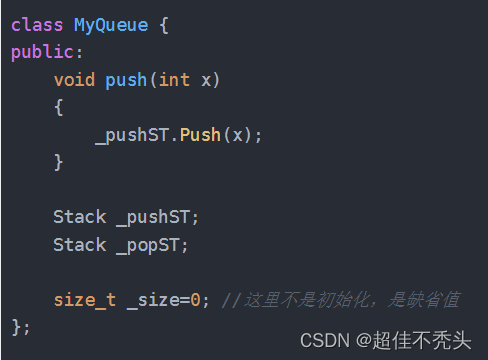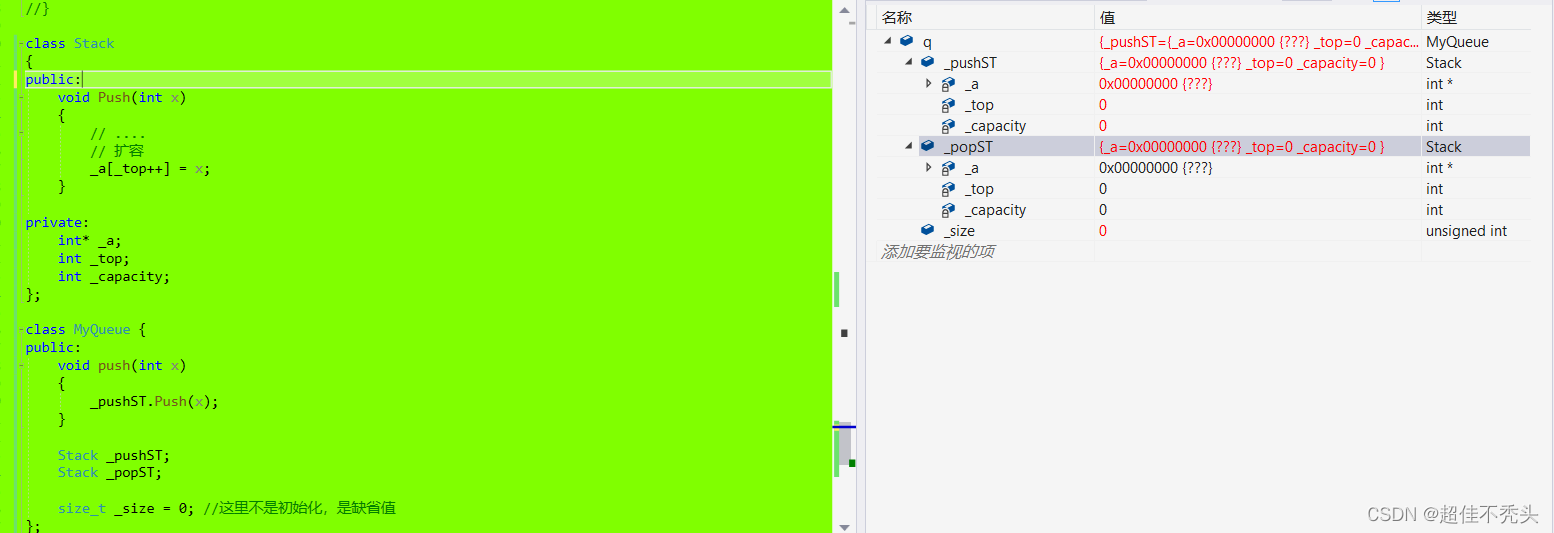可以看出，默认提供的并没有将数组开辟空间，不能满足我们的需求。

## 3.析构函数

### 2.特性

1. 析构函数名是在类名前加上字符 ~ 。（~这个符号在C语言里面是二进制取反）
2. 无参数无返回值类型。
3. 一个类只能有一个析构函数。若未显式定义，系统会自动生成默认的析构函数。 注意：析构

4. 对象 生命周期 结束时， C++ 编译系统系统自动调用析构函数。
析构函数在对象生命周期结束时自动调用，生命周期得具体看，如果在局部域（函数内部），则函数调用完毕后，就会结束局部变量的生命周期，全局域，malloc（手动结束）。
``````class Stack
{
public:

//析构函数名是在类名前加上字符 ~
~Stack()
{
free(_a);
_a=nullptr;
_top=_capacity=0;
}

private:
int* _a;
int _top;
int _capacity;
};
``````

## 4.拷贝构造函数

### 1.概念

``````class Date
{
public:
//构造函数
Date(int year = 1900, int month = 1, int day = 1)
{
_year = year;
_month = month;
_day = day;
}

void print()
{
cout << _year << " " << _month << " " << _day << endl;
}
private:
int _year;
int _month;
int _day;
};

int main()
{
Date d1(2022, 10, 10);
Date d2(d1); //拷贝d1
d1.print();
d2.print();
return 0;
}
``````

### 2.特性

1. 拷贝构造函数是构造函数的一个重载形式。函数名相同，参数不同。

2. 拷贝构造函数的参数只有一个且必须是类类型对象的引用，使用传值方式编译器直接报错，因为会引发无穷递归调用。

Date(Date d)  //去掉引用，就是传值调用
{
_year = d._year;
_month = d._month;
_day = d._day;
}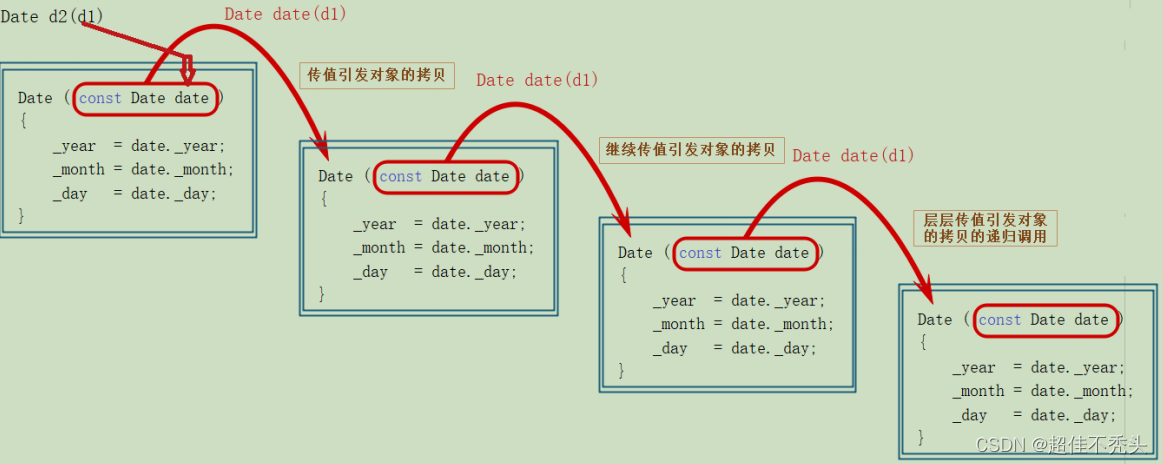Date(Date *d)  //去掉引用，就是传值调用
{
_year = d->_year;
_month = d->_month;
_day = d->_day;
}

3.拷贝构造函数只有单个形参，该形参是对本类类型对象的引用，一般常用const修饰

Date(const  Date & d)  //去掉引用，就是传值调用
{
_year = d._year;

d._month=_month ; //有时候会写反，而改变了原本对象的值。
_day = d._day;
}

Date d2(d1); //拷贝d1

4. 若未显示定义，编译器会生成默认的拷贝构造函数。 默认的拷贝构造函数对象按内存存储按

``````class Date
{
public:
//构造函数
Date(int year = 1900, int month = 1, int day = 1)
{
cout << "构造函数" << endl; //验证调用所需
_year = year;
_month = month;
_day = day;
}

private:
int _year;
int _month;
int _day;
};

int main()
{
Date d1(2022, 10, 10);
Date d2(d1); //拷贝d1
return 0;
}
``````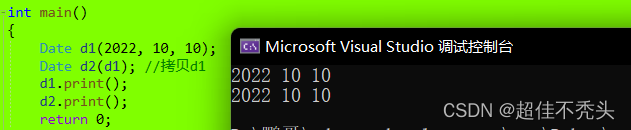``````class Stack
{
public:
Stack(int capacity = 4)
{
cout << "Stack(int capacity = 4)" << endl;

_a = (int*)malloc(sizeof(int)*capacity);
if (_a == nullptr)
{
perror("malloc fail");
exit(-1);
}

_top = 0;
_capacity = capacity;
}
//析构函数
~Stack()
{
cout << "~Stack()" << endl;

free(_a);
_a = nullptr;
_top = _capacity = 0;
}

void Push(int x)
{
// ....
// 扩容
_a[_top++] = x;
}

private:
int* _a;
int _top;
int _capacity;
};

int main()
{
Stack s1;
s1.Push(1);
s1.Push(2);
Stack s2(s1);
}``````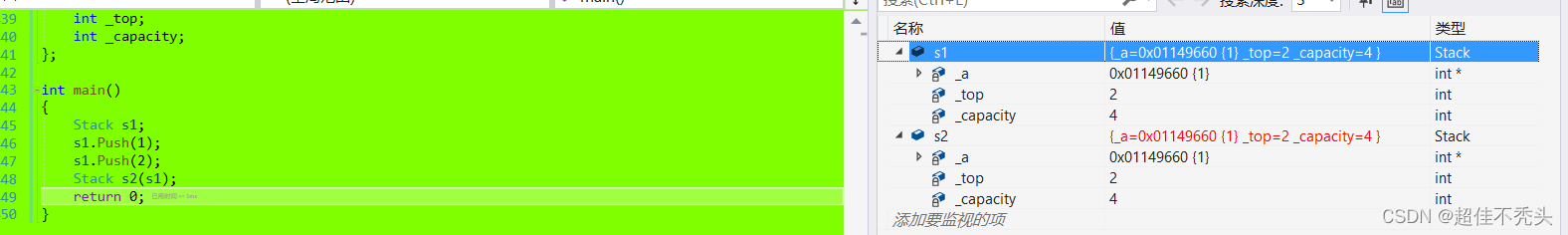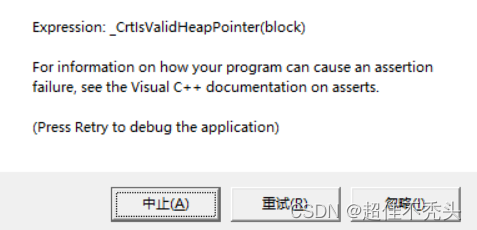这是怎么了？？编译器自己提供的默认拷贝构造函数居然把s1拷贝到s2类中时，两个类中的指针变量指向了同一块空间！！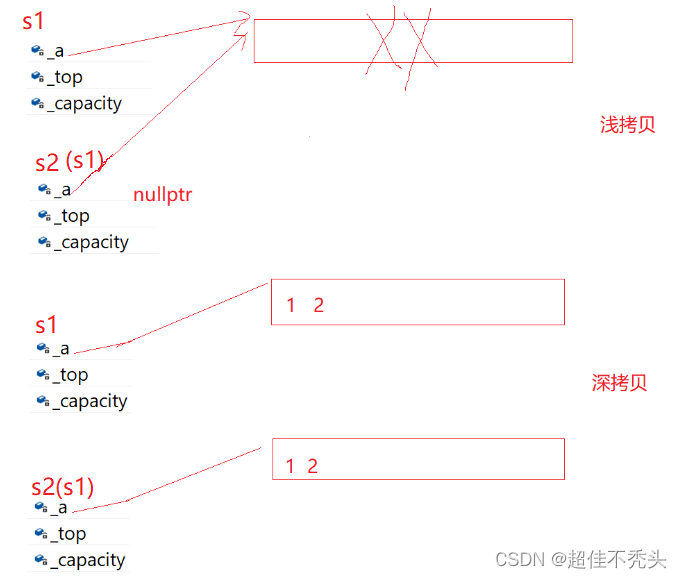``````//st2(st1)拷贝构造
Stack(const Stack& st)
{
cout << "Stack(const Stack& st)" << endl;

_a = (int*)malloc(sizeof(int)*st._capacity);
if (_a == nullptr)
{
perror("malloc fail");
exit(-1);
}
memcpy(_a, st._a, sizeof(int)*st._top);
_top = st._top;
_capacity = st._capacity;
}``````

即解决了指针重复指向一块空间，又把已经创建好的s1对象和其中的内容都拷贝到了s2。总结：需要写析构函数的类，都需要写深拷贝的拷贝构造，如：Stack

## 5.运算符重载

### 2.赋值运算符重载格式

``````class Data
{
public:
Data(int year=1,int month=1,int day=1)
{
_year = year;
_month = month;
_day = day;
}
private:
int _year;
int _month;
int _day;
};

bool operator==(Data& d1，Data& d2)
{
return d1._year == d2._year
&& d1._month == d2._month
&& d1._day == d2._day;
}

int main()
{
Data d1(2022, 10, 11);
Data d2(2023, 1, 1);
d1==d2;//可以显式调用 operator==(d1,d2);
cout << (d1 == d2) << endl;
cout << (d1 > d2) << endl;
}``````

1.定义运算符重载时，参数为引用的好处是，不需要调用拷贝函数，若是传值,参数为对象，则需要拷贝对象，调用拷贝函数。

2.定义在类外，需要将类的成员变量设置为公开，会造正麻烦，那么我们直接就定义到了类的内部。

3.若直接放入类的内部定义运算符重载，则会出现问题：

``````class Data
{
public:
Data(int year=1,int month=1,int day=1)
{
_year = year;
_month = month;
_day = day;
}

bool operator==(Data& d)
{
return this->_year == d2._year
&& this->_month == d2._month
&& this->_day == d2._day;
}

private:
int _year;
int _month;
int _day;
};

int main()
{
Data d1(2022, 10, 11);
Data d2(2023, 1, 1);
d1==d2;//可以显式调用 operator==(d1,d2);
cout << (d1 == d2) << endl;
cout << (d1 > d2) << endl;
}``````

bool operator==(Data& d)
{
return this->_year == d2._year
&& this->_month == d2._month
&& this->_day == d2._day;
}

&& this->_month == d2._month
&& this->_day == d2._day;

### 3.加法的实现

``````#define _CRT_SECURE_NO_WARNINGS 1
#include<iostream>
using namespace std;

class Data
{
public:
Data(int year=1,int month=1,int day=1)
{
_year = year;
_month = month;
_day = day;
}

int Getmonthday(int year, int month)
{
int a = { 0,31,28,31,30,31,30,31,31,30,31,30,31 };
if (month == 2 && (year % 4 == 0 && year % 100 != 0 || year % 4 == 0))
{
return 29;
}
else
return a[month];
}

Data& operator+=(int day)
{
_day += day;
while (_day> Getmonthday(this->_year, this->_month))
{
_day -= Getmonthday(this->_year, this->_month);
_month++;
if (_month > 12)
{
_month -= 12;
_year++;
}
}
cout << _year<<_month<<_day << endl;
return *this;
}

Data operator+(int day)
{
Data ret(*this);
ret +=day;
return ret;
}

private:
int _year;
int _month;
int _day;
};
int main()
{
Data d1(2022, 10, 11);
Data d2(2023, 1, 1);
d1 + 100;
d1 += 100;
return 0;
}``````

## 6.赋值运算符重载

### 1.赋值运算符重载格式

``````Date& operator=(const Date& d)
{
if (this != &d)
{
_year = d._year;
_month = d._month;
_day = d._day;
}
return *this;
}

d1=d2;``````

### 2.拷贝构造和赋值重载

d1=d2;就是把d2的值赋值给d1，那么是不是和拷贝构造也有相似之处呢？？

``````class Stack
{
public:
Stack(int capacity = 4)
{
cout << "Stack(int capacity = )" <<capacity<<endl;

_a = (int*)malloc(sizeof(int)*capacity);
if (_a == nullptr)
{
perror("malloc fail");
exit(-1);
}

_top = 0;
_capacity = capacity;
}

// st2(st1)
Stack(const Stack& st)
{
cout << "Stack(const Stack& st)" << endl;

_a = (int*)malloc(sizeof(int)*st._capacity);
if (_a == nullptr)
{
perror("malloc fail");
exit(-1);
}
memcpy(_a, st._a, sizeof(int)*st._top);
_top = st._top;
_capacity = st._capacity;
}

~Stack()
{
cout << "~Stack()" << endl;

free(_a);
_a = nullptr;
_top = _capacity = 0;
}

void Push(int x)
{
// ....
// 扩容
_a[_top++] = x;
}

private:
int* _a;
int _top;
int _capacity;
};

int main()
{
Stack st1;
st1.Push(1);
Stack st2(10);
st2.Push(1);
st2.Push(2);
st2.Push(3);
st1 = st2;
return 0;
}``````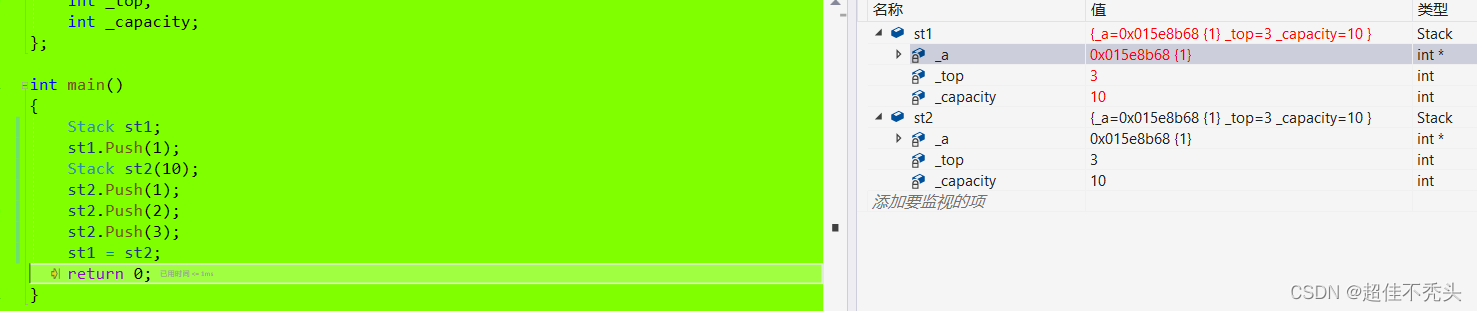会发现和之前的拷贝构造出现的问题挺一样的，d1和d2的_a指向的空间是一样的，结果同一块空间还析构了两次，d1原本指向的空间，没人析构，造成内存泄露。当要调用析构函数时，就需要我们自己去写。

``````Stack& operator=(const Stack& st)
{
if (this != &st)
{
free(_a);
_a = (int*)malloc(sizeof(int) * st._capacity);
if (_a == nullptr)
{
perror("malloc fail");
exit(-1);
}
memcpy(_a, st._a, sizeof(int) * st._top);
_top = st._top;
_capacity = st._capacity;
}

return *this;
}``````

## 7.<<和>>运算符重载

<<在C语言中是左移的意思，但在c++中却是流插入的意思。

int i=5;

cout<<i;   <<流插入运算符，这个运算符支持内置类型（int,double,char......)，是因为他在库中就已经实现了,而cout能用<<,是因为cout的类中也已经实现了使用流插入的功能。

cin和cout在库中对应的类是：istream,ostream，都已经支持自定义类型。

``````void operator<<(ostream& out)
{
out << _year << " " << _month << " " << _day << endl;
}``````

d1<<cout;但是这样总是很怪，流插入不应该流向cout吗？那么我们放在类外依旧在.h中，写成全局函数试试：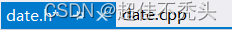``````void operator<<(const ostream& out, const Date& d)
{
out << _year << " " << _month << " " << _day << endl;
}``````

1.static修饰函数，会改变链接属性（是在当前文件可见），在其他文件不会产生符号表。那么不产生符号表，直接就会使用定义，不会发生冲突。

2.声明和定义分离 .h中声明，.cpp中定义，就不会发生符号表重复。

3.加inline内联，加了内联，就不会产生符号表，也是直接展开，定义到了每个有.h的文件，直接使用定义，不会链接。void operator<<(const ostream&  out, const Date&  d);

1.偷家，私有成员变量不可以用，但我可以通过公有的成员函数去使用。

2.友元：友元声明（在类和对象下我们会详细说明），在类中的声明前加一个friend.

friend void operator<<(const ostream&  out, const Date&  d);

ostream& operator<<(const ostream&  out, const Date&  d);   你懂了吗？？## 8.const修饰的成员函数

### 1.const修饰的权限问题

1.举例说明：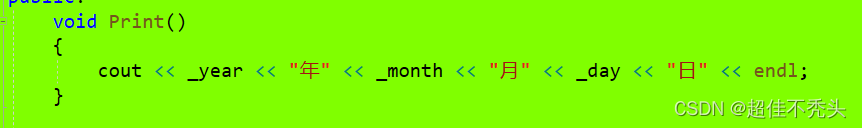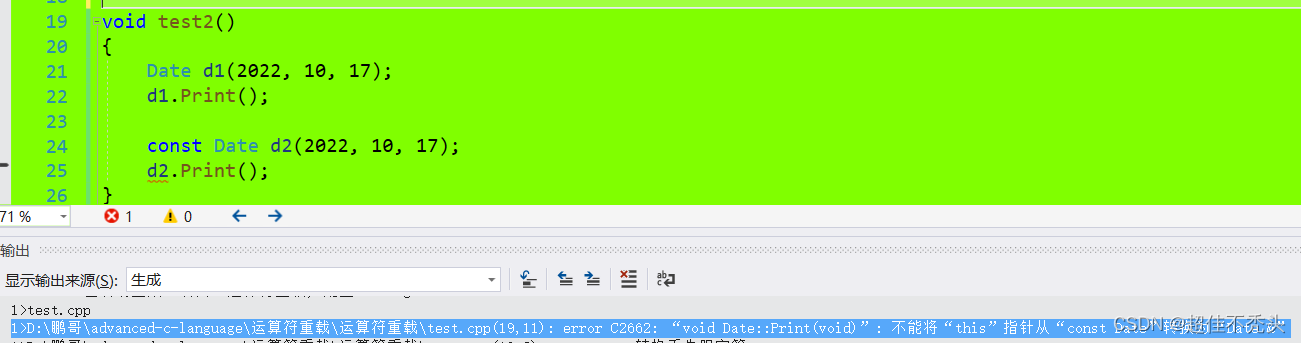为什么就会报错呢？？当d2去调用的时候&d2（即const Date*)，这里const修饰的是指针所指的对象不可以被修改，只读。但是传参的时候就成了Print(Date*const this)，可读可写（不明白可以回顾以前文章）。所以扩大了权限，无法调用。

2.举例说明：

int Date:: operator-(const Date& d)
{
Date max = *this;
Date min = d;
int flag = 1;
//if (*this < d)

换为

if(d>*this)  可以吗？？  当然不可以，d.operator>(*this);
{                                   &d ->(const Date*)d此时是只读，结果到了>运算符的参数就是

Date*const可读可写，权限放大。
max = d;
min = *this;
flag = -1;
}
int count = 0;
while (max != min)
{
max--;
count++;
}
return count * flag;;
}

### 2.取地址重载

就是我不想让他取出地址：

``````    Date* operator&()
{
return nullptr;
//return this;
}

//第二种返回值加const是因为，返回参数是this时，为了返回时不扩大权限
const Date* operator&() const
{
return nullptr;
//return this;
}``````

# 总结